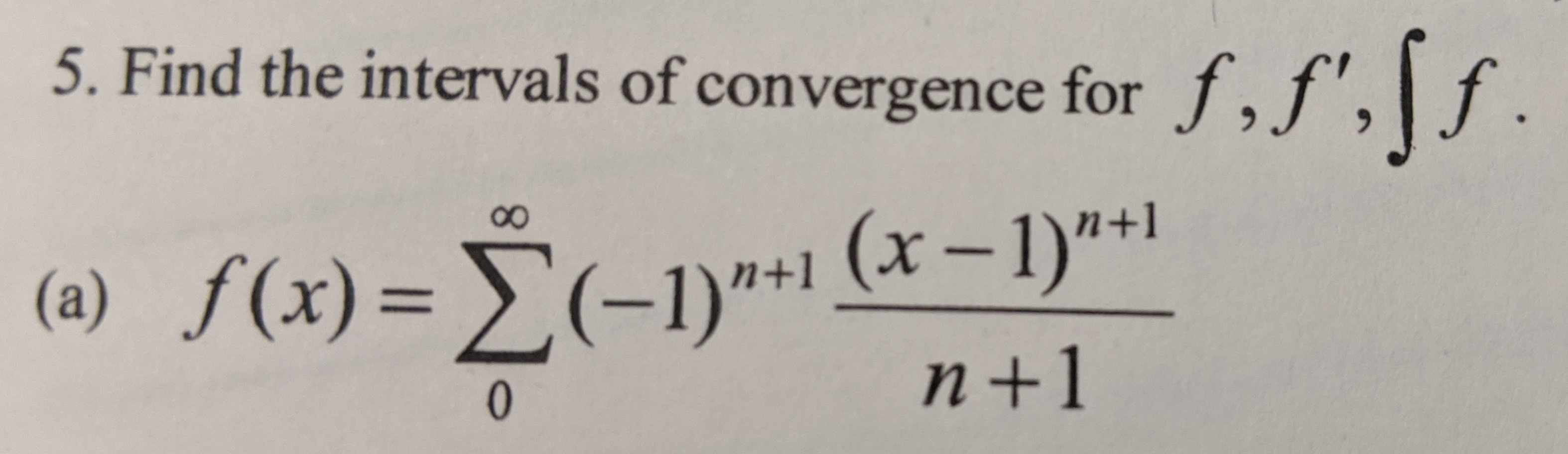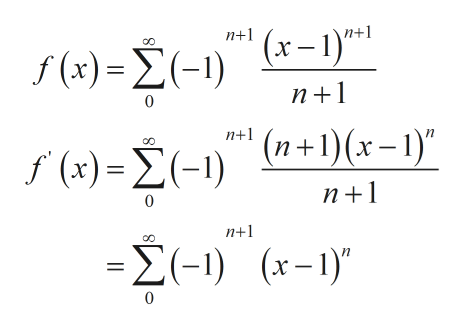# 5. Find the intervals of convergence for f,f',\f.f(x) = (-1)"+1 (x– 1)"+1n+18.(a) ƒ(x)= E(-1)"*1%3D0.

Question
177 views

I need help trying to find the interval of convergence for the derivative of the serieshelp_outlineImage Transcriptionclose5. Find the intervals of convergence for f,f',\f . f(x) = (-1)"+1 (x– 1)"+1 n+1 8. (a) ƒ(x)= E(-1)"*1 %3D 0. fullscreen
check_circle

Step 1

For the given series first find the derivative of ‘f’ in order to find the interval of convergence for the first derivative of ‘f’. The derivative is calculated as.help_outlineImage Transcriptionclosen+1 (x-1)*1 n+1 f() - Σ-)κ-) п+1 (n+1)(x– 1)" n+1 f (x)= É(-1) n+1 n+1 -Σ-1) ( - 1), fullscreen
Step 2

The nth term of this serie...

### Want to see the full answer?

See Solution

#### Want to see this answer and more?

Solutions are written by subject experts who are available 24/7. Questions are typically answered within 1 hour.*

See Solution
*Response times may vary by subject and question.
Tagged in

### Calculus